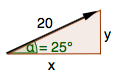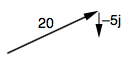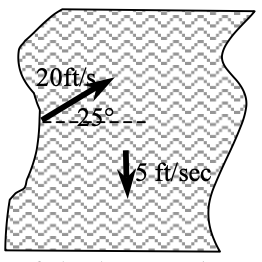### Home > PC > Chapter 10 > Lesson 10.1.2 > Problem10-25

10-25.

A boat is headed up a river at an angle of $25°$ with a rate of $20$ feet per second. The current is heading down stream at a rate of $5$ feet per second. The river is $100$ feet wide.

1. Express the velocity of the boat as a vector in component form without including the effect of the river.2. The actual motion of the boat is the combination of the motion of the boat and the current in the river. Find the vector that gives the actual motion of the boat.

Use vector addition to find the resultant vector.3. How long will it take the boat to cross the river?

Consider the 'i' component of the resultant vector from part (b).

4. How far up river will the boat arrive?

Consider the 'j' component of the resultant vector from part (b). Multiply this by the number of seconds found in part (c).

$19.044$ feet